Prime Factorization MathBitsNotebook.com Terms of Use   Contact Person: Donna Roberts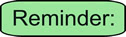Integers:    ... -5, -4, -3, -2, -1, 0, 1, 2, 3, 4, 5, ... (no fractions or decimals)

 Prime numbers are integers (greater than 1) that can be divided exactly by only 1 or itself.
Prime Numbers:
2, 3, 5, 7, 11, 13, 17, 19, 23, 29, 31, 37, 41, 43, 47, 53, 59. 61, 67, 71, 73, 79, 83, 89, 97, ...

Positive integers that are not prime numbers are called composite numbers.
 A composite number is a positive integer that has more factors than just 1 and itself. Examples of composite numbers are: 4, 6, 8, 9 10, 12, 14, 15, 16, 18, 20, 21, ... The number 24 is an example of a composite number. Its factors are 1, 2, 3, 4, 6, 8, 12, and 24. All of these factors divide exactly into 24.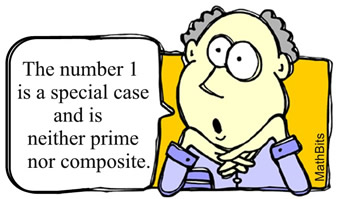Factors are numbers you multiply to get another number.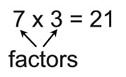Prime factorization is the process of finding only prime numbers that will multiply together to form a starting number. It breaks a number down into its prime factors.

This process of prime factorization is based upon the Fundamental Theorem of Arithmetic: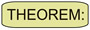Fundamental Theorem of Arithmetic Any integer greater than 1 is either a prime number, or can be written as a unique product of prime numbers. (The order of the prime numbers is ignored.)
 This basically means that every integer greater than one, that is not prime, can be written as the multiplication (the product) of only prime numbers. And there is only one such set of primes per number.Examples: While you can start your factoring with any prime number that will divide exactly into your number, starting with the smaller prime numbers is usually the easiest process. The diagrams shown below with the segments, are called factor trees.

1. Write 12 as the product of primes: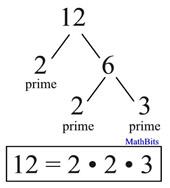• 12 was divided by the small prime 2
• 6 was also divide by 2
• since 3 is prime, the process is done

• the answer can be written with exponents
12 = 22 • 3

2. What are the prime factors of 48?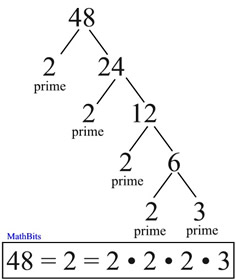48 = 24 • 3
3a. Write 75 as the product of primes: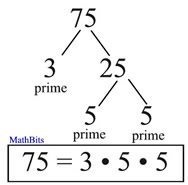75 = 3 • 52
3b. Write 75 as the product of primes:
This is a short-hand version of the factor tree. It shows the primes and the division. Notice that the primes appear on the left hand side.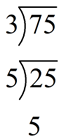4. What is the prime factorization of 55?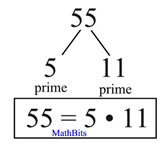If you are having a hard time finding a prime that will divide into your number, you can start with any factors of the number that may be more obvious.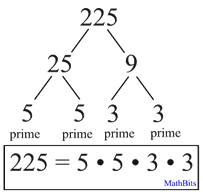225 = 32 • 52Prime factorization can be used to reduce fractions.
(This process will always get you the simplest form of the fraction.)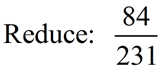Solution using prime factorization: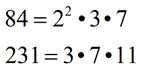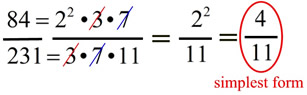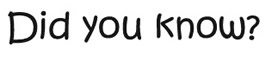• Every prime factor of a perfect square appears an even number of times. (The exponents will be even numbers.)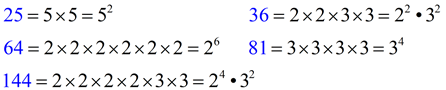• A number is called a perfect number if it is equal to the sum of its positive divisors excluding itself. Consider 6: its factors are 1, 2, 3 and 6 1 + 2 + 3 = 6 making 6 a perfect number. Consider 28: its factors are 1, 2, 4, 7, 14, 28 1 + 2 + 4 + 7 + 14 = 28 making 28 a perfect number.